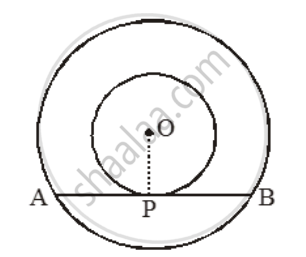# Prove that in Two Concentric Circles, the Chord of the Larger Circle Which Touches the Smaller Circle, is Bisected at the Point of Contact. - Mathematics

Sum

Prove that in two concentric circles, the chord of the larger circle which touches the smaller circle, is bisected at the point of contact.

#### Solution

Given: Two circles with the same centre O and AB is a chord of the larger circle which touches the smaller circle at P.To prove: AP = BP.

Construction: Join OP.

Proof: AB is a tangent to the smaller circle at the point P and OP is the radius through P.

∴ OP ⊥ AB.

But, the perpendicular drawn from the centre of a circle to a chord bisects the chord.

∴ OP bisects AB. Hence, AP = BP

Concept: Concept of Circle - Centre, Radius, Diameter, Arc, Sector, Chord, Segment, Semicircle, Circumference, Interior and Exterior, Concentric Circles
Is there an error in this question or solution?